# Part 2 : tf.keras Convolutional API

Ideal read would http://cs231n.github.io/convolutional-networks/#overview to get the full understanding on the Convolutional network.

Convolution Neural Network is a type of feed forward neural network that is generally used for Image Recognition and Image Classification tasks.

There are four layers in a convolution neural network. These are Convolution layer, ReLU layer, Pooling layer and Fully Connected Layer.

The 3rd layer is called the Pooling layer. It is used to reduce the dimensionality of the feature map. The output is a pooled featured map.Pooling layers uses different filters to identify different parts of the image like edges, corners, body, etc.

The pooled feature map is then converted into a long continuous linear vector. This process is called Flattening. This flattened matrix goes through a Fully Connected Layer to classify the images.In today’s tutorial, we are going to discuss the Keras Conv2D class, including the most important parameters you need to tune when training your own Convolutional Neural Networks (CNNs). From there we are going to use the Keras Conv2D class to implement a simple CNN. We’ll then train and evaluate this CNN on the CALTECH-101 dataset.

The inspiration for today’s post came after Tensorflow 2.0 release, where KEras APIs became the mainstream APIs.

In today’s tutorial we are going to discuss each of the parameters to the Keras Conv2D class, explain each one, and provide examples of situations where and when you would want to set specific values, enabling you to:

1. Quickly determine if you need to utilize a specific parameter to the Keras Conv2D class
2. Decide on a proper value for that specific parameter
3. Effectively train your own Convolutional Neural Network

## Keras Conv2D and Convolutional Layers

In the first part of this tutorial, we are going to discuss the parameters to the Keras Conv2D class. From there we are going to utilize the Conv2D class to implement a simple Convolutional Neural Network. We’ll then take our CNN implementation and then train it on the CALTECH-101 dataset. Finally, we’ll evaluate the network and examine its performance. Let’s go ahead and get started!

### Conv2D

The Keras Conv2D class constructor has the following signature:

[/crayon]
Looks a bit overwhelming, right?

How in the world are you supposed to properly set these values?

No worries — let’s examine each of these parameters individually, giving you a strong understanding of not only what each parameter controls but also how to properly set each parameter as well.

#### 1.filters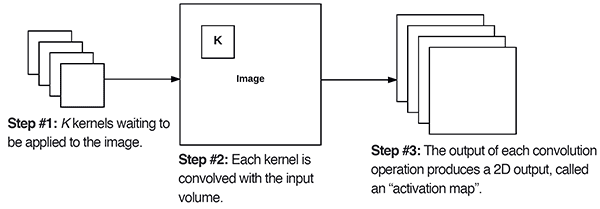Figure 1: The Keras Conv2D parameter,

determines the number of kernels to convolve with the input volume. Each of these operations produces a 2D activation map.

The first required Conv2D parameter is the number of filters  that the convolutional layer will learn.

Layers early in the network architecture (i.e., closer to the actual input image) learn fewer convolutional filters while layers deeper in the network (i.e., closer to the output predictions) will learn more filters.

Lets take a side track and learn what is Max Pooling:Here in the figure, we show the operation upon the pixel space. Alternatively we can do a similar operation on some other mathematical space. Also, one can change the operation of taking ‘Max’ to something else, say taking an ‘Average’ (This is what is done in Average Pooling).

Conv2D layers in between will learn more filters than the early Conv2D layers but fewer filters than the layers closer to the output. Let’s go ahead and take a look at an example:

On Line 1 we learn a total of 32  filters. Max pooling is then used to reduce the spatial dimensions of the output volume.

We then learn 64  filters on Line 4. Again max pooling is used to reduce the spatial dimensions.

The final Conv2D layer learns 128  filters.

Notice at as our output spatial volume is decreasing our number of filters learned is increasing — this is a common practice in designing CNN architectures and one I recommend you do as well. As far as choosing the appropriate number of filters , I nearly always recommend using powers of 2 as the values.

You may need to tune the exact value depending on (1) the complexity of your dataset and (2) the depth of your neural network, but I recommend starting with filters in the range [32, 64, 128] in the earlier and increasing up to [256, 512, 1024] in the deeper layers.

Again, the exact range of the values may be different for you, but start with a smaller number of filters and only increase when necessary.

#### 2.kernel_size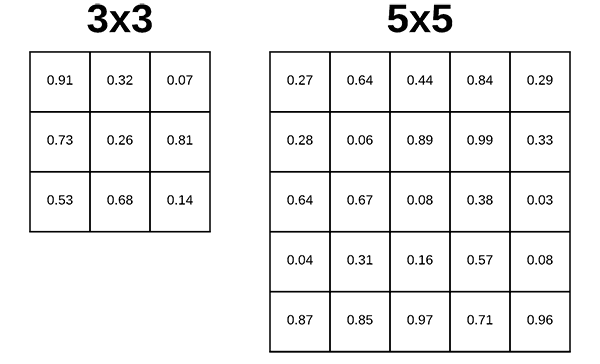Figure 2: The Keras deep learning Conv2D parameter,

, determines the dimensions of the kernel. Common dimensions include 1×1, 3×3, 5×5, and 7×7 which can be passed as

,

,

, or

tuples.

The second required parameter you need to provide to the Keras Conv2D class is the kernel_size , a 2-tuple specifying the width and height of the 2D convolution window.

The kernel_size  must be an odd integer as well.

Typical values for kernel_size  include: (1, 1) , (3, 3) , (5, 5) , (7, 7) . It’s rare to see kernel sizes larger than 7×7.

So, when do you use each?

If your input images are greater than 128×128 you may choose to use a kernel size > 3 to help (1) learn larger spatial filters and (2) to help reduce volume size.

Other networks, such as VGGNet, exclusively use (3, 3)  filters throughout the entire network.

More advanced architectures such as Inception, ResNet, and SqueezeNet design entire micro-architectures which are “modules” inside the network that learn local features at different scales (i.e., 1×1, 3×3, and 5×5) and then combine the outputs.

A great example can be seen in the Inception module below: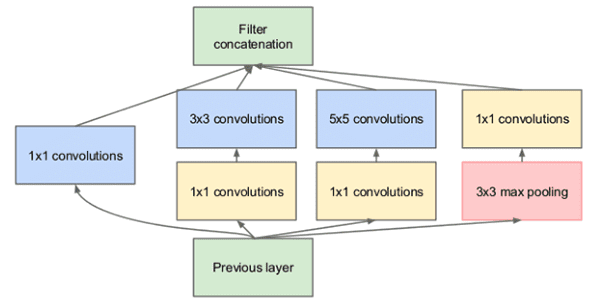Figure 3: The Inception/GoogLeNet CNN architecture uses “micro-architecture” modules inside the network that learn local features at different scales (

) and then combine the outputs.

The Residual module in the ResNet architecture uses 1×1 and 3×3 filters as a form of dimensionality reduction which helps to keep the number of parameters in the network low (or as low as possible given the depth of the network):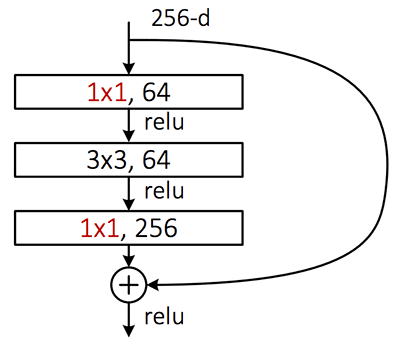Figure 4: The ResNet “Residual module” uses 1×1 and 3×3 filters for dimensionality reduction. This helps keep the overall network smaller with fewer parameters.

So, how should you choose your filter_size ?

First, examine your input image — is it larger than 128×128?

If so, consider using a 5×5 or 7×7 kernel to learn larger features and then quickly reduce spatial dimensions — then start working with 3×3 kernels:

If your images are smaller than 128×128 you may want to consider sticking with strictly 1×1 and 3×3 filters.

And if you intend on using ResNet or Inception-like modules you’ll want to implement the associated modules and architectures by hand. Covering how to implement these modules is outside the scope of this tutorial.

#### 3.strides

The strides  parameter is a 2-tuple of integers, specifying the “step” of the convolution along the x and y axis of the input volume.

The strides  value defaults to (1, 1) , implying that:

1. A given convolutional filter is applied to the current location of the input volume
2. The filter takes a 1-pixel step to the right and again the filter is applied to the input volume
3. This process is performed until we reach the far-right border of the volume in which we move our filter one pixel down and then start again from the far left

Typically you’ll leave the strides  parameter with the default (1, 1)  value; however, you may occasionally increase it to (2, 2)  to help reduce the size of the output volume (since the step size of the filter is larger).

Typically you’ll see strides of 2×2 as a replacement to max pooling:

Here we can see our first two Conv2D layers have a stride of 1×1. The final Conv2D layer; however, takes the place of a max pooling layer, and instead reduces the spatial dimensions of the output volume via strided convolution.

In 2014, Springenber et al. published a paper entitled Striving for Simplicity: The All Convolutional Net which demonstrated that replacing pooling layers with strided convolutions can increase accuracy in some situations.

ResNet, a popular CNN, has embraced this finding — if you ever look at the source code to a ResNet implementation (or implement it yourself), you’ll see that ResNet replies on strided convolution rather than max pooling to reduce spatial dimensions in between residual modules.Figure 5: A 3×3 kernel applied to an image with padding. The Keras Conv2D padding parameter accepts either

(padding + preserving spatial dimensions). This animation was contributed to StackOverflow (source).

The padding  parameter to the Keras Conv2D class can take on one of two values: valid  or same .

With the valid  parameter the input volume is not zero-padded and the spatial dimensions are allowed to reduce via the natural application of convolution.

The following example would naturally reduce the spatial dimensions of our volume:

Note: See this tutorial on the basics of convolution if you need help understanding how and why spatial dimensions naturally reduce when applying convolutions.

If you instead want to preserve the spatial dimensions of the volume such that the output volume size matches the input volume size, then you would want to supply a value of same  for the padding :

While the default Keras Conv2D value is valid  I will typically set it to same  for the majority of the layers in my network and then either reduce spatial dimensions of my volume by either:

1. Max pooling
2. Strided convolution

I would recommend that you use a similar approach to padding with the Keras Conv2D class as well.

#### 5.data_format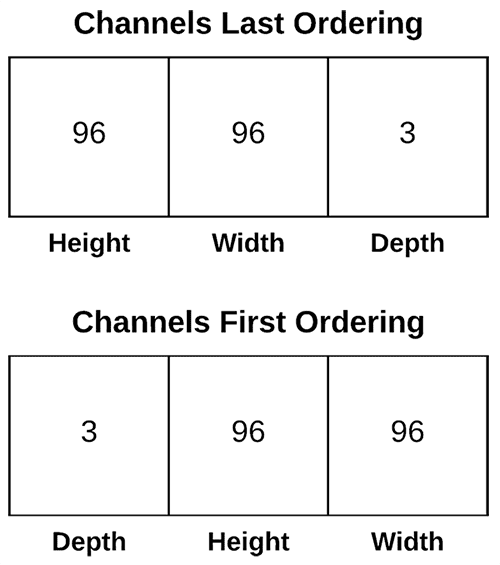Figure 6: Keras, as a high-level framework, supports multiple deep learning backends. Thus, it includes support for both “channels last” and “channels first” channel ordering.

The data format value in the Conv2D class can be either channels_last  or channels_first :

• The TensorFlow backend to Keras uses channels last ordering.
• The Theano backend uses channels first ordering.

You typically shouldn’t have to ever touch this value as Keras for two reasons:

1. You are more than likely using the TensorFlow backend to Keras
2. And if not, you’ve likely already updated your ~/.keras/keras.json  configuration file to set your backend and associated channel ordering

My advice is to never explicitly set the data_format  in your Conv2D class unless you have a very good reason to do so.

#### 6.dilation_rate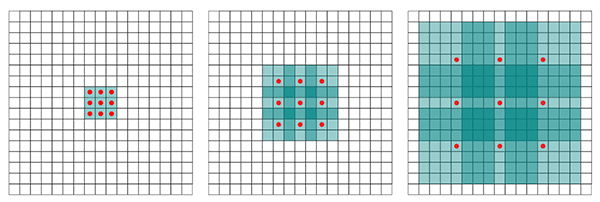Figure 7: The Keras deep learning Conv2D parameter,

, accepts a 2-tuple of integers to control dilated convolution (source).

The dilation_rate  parameter of the Conv2D class is a 2-tuple of integers, controlling the dilation rate for dilated convolution. Dilated convolution is a basic convolution only applied to the input volume with defined gaps, as Figure 7 above demonstrates.

You may use dilated convolution when:

1. You are working with higher resolution images but fine-grained details are still important
2. You are constructing a network with fewer parameters

Discussing dilated convolution is outside the scope of this tutorial so if you are interested in learning more, please refer to this tutorial.

#### 7.activation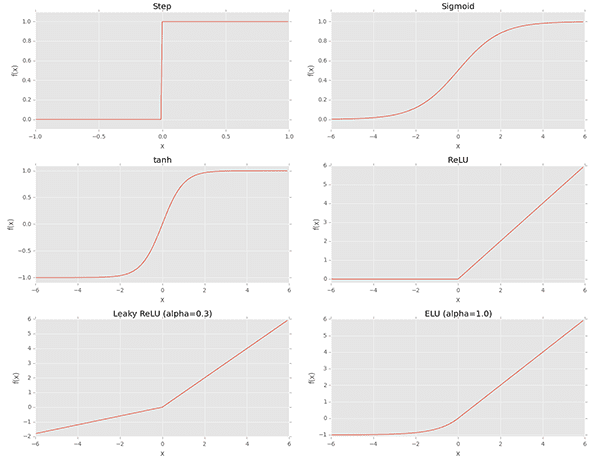Figure 8: Keras provides a number of common activation functions. The

parameter to Conv2D is a matter of convenience and allows the activation function for use after convolution to be specified.

The activation  parameter to the Conv2D class is simply a convenience parameter, allowing you to supply a string specifying the name of the activation function you want to apply after performing the convolution.

In the following example we perform convolution and then apply a ReLU activation function:

Use the activation  parameter if you and if it helps keep your code cleaner — it’s entirely up to you and won’t have an impact on the performance of your Convolutional Neural Network.

#### 8.use_bias

The use_bias  parameter of the Conv2D class controls whether a bias vector is added to the convolutional layer.

Typically you’ll want to leave this value as True , although some implementations of ResNet will leave the bias parameter out.

I recommend keep the bias unless you have a good reason not to.

#### 9.kernel_initializer and bias_initializer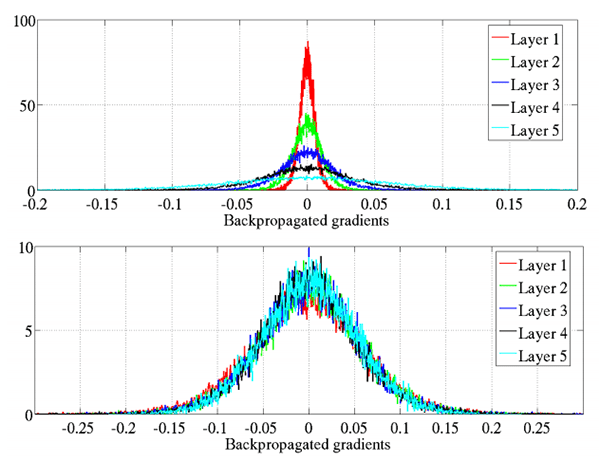Figure 9: Keras offers a number of initializers for the Conv2D class. Initializers can be used to help train deeper neural networks more effectively.

The kernel_initializer  controls the initialization method used to initialize all values in the Conv2D class prior to actually training the network.

Similarly, the bias_initializer  controls how the bias vector is initialized before training starts.

A full list of initializers can be found in the Keras documentation; however, here is what I recommend:

1. Leave the bias_initialization  alone — it will by default filled with zeros (you’ll rarely if ever, have to change the bias initialization method.
2. The kernel_initializer  defaults to glorot_uniform , the Xavier Glorot uniform initialization method, which is perfectly fine for the majority of tasks; however, for deeper neural networks you may want to use  he_normal  (MSRA/He et al. initialization) which works especially well when your network has a large number of parameters (i.e., VGGNet).

In the vast majority of CNNs we implement we either choose glorot_uniform  or he_normal  — we recommend you do the same unless you have a specific reason to use a different initializer.

#### 10.kernel_regularizer, bias_regularizer, and activity_regularizer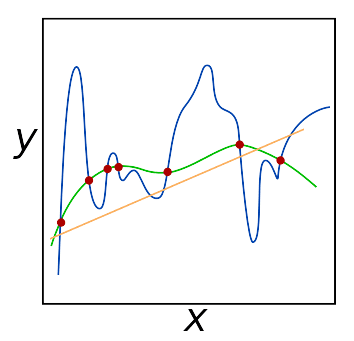Figure 10: Regularization hyperparameters should be adjusted especially when working with large datasets and really deep networks. The kernel_regularizer parameter in particular is one that I adjust often to reduce overfitting and increase the ability for a model to generalize to unfamiliar images.

The kernel_regularizer , bias_regularizer , and activity_regularizer  control the type and amount of regularization method applied to the Conv2D layer.

Applying regularization helps you to:

1. Reduce the effects of overfitting
2. Increase the ability of your model to generalize

When working with large datasets and deep neural networks applying regularization is typically a must.

Normally you’ll encounter either L1 or L2 regularization being applied — I will use L2 regularization on my networks if I detect signs of overfitting:

from keras.regularizers import l2

kernel_regularizer=l2(0.0005))

The amount of regularization you apply is a hyperparameter you will need to tune for your own dataset, but I find values of 0.0001-0.001 are good ranges to start with.

We would suggest leaving your bias regularizer alone — regularizing the bias typically has very little impact on reducing overfitting.

We also suggest leaving the activity_regularizer  at its default value (i.e., no activity regularization).

While weight regularization methods operate on weights themselves, f(W), where f is the activation function and W are the weights, an activity regularizer instead operates on the outputs, f(O), where O is the outputs of a layer.

Unless there is a very specific reason you’re looking to regularize the output it’s best to leave this parameter alone.

#### 11.kernel_constraint and bias_constraint

The final two parameters to the Keras Conv2D class are the kernel_constraint  and bias_constraint .

These parameters allow you to impose constraints on the Conv2D layer, including non-negativity, unit normalization, and min-max normalization.

You can see the full list of supported constraints in the Keras documentation.

Again, I would recommend leaving both the kernel constraint and bias constraint alone unless you have a specific reason to impose constraints on the Conv2D layer.

Check out the repo @ https://github.com/dhiraa/medium/tree/master/keras_conv_net_basics for working version of the code!